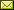## Model-Checking Process Equivalences

 Martin Lange (University of Kassel) Etienne Lozes (University of Kassel) Manuel Vargas Guzmán (University of Kassel)

 Process equivalences are formal methods that relate programs and system which, informally, behave in the same way. Since there is no unique notion of what it means for two dynamic systems to display the same behaviour there are a multitude of formal process equivalences, ranging from bisimulation to trace equivalence, categorised in the linear-time branching-time spectrum. We present a logical framework based on an expressive modal fixpoint logic which is capable of defining many process equivalence relations: for each such equivalence there is a fixed formula which is satisfied by a pair of processes if and only if they are equivalent with respect to this relation. We explain how to do model checking, even symbolically, for a significant fragment of this logic that captures many process equivalences. This allows model checking technology to be used for process equivalence checking. We show how partial evaluation can be used to obtain decision procedures for process equivalences from the generic model checking scheme.

In Marco Faella and Aniello Murano: Proceedings Third International Symposium on Games, Automata, Logics and Formal Verification (GandALF 2012), Napoli, Italy, September 6-8, 2012, Electronic Proceedings in Theoretical Computer Science 96, pp. 43–56.
Published: 7th October 2012.

 ArXived at: http://dx.doi.org/10.4204/EPTCS.96.4 bibtex PDF
References in reconstructed bibtex, XML and HTML format (approximated).
 Comments and questions to:eptcs@eptcs.org For website issues:webmaster@eptcs.org